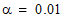# NMath User's Guide

45.1 One Sample Kolmogorov-Smirnov Test (.NET, C#, CSharp, VB, Visual Basic, F#)

Class performs a Kolmogorov-Smirnov test of the distribution of one sample. This class compares the distribution of a given sample to the hypothesized distribution defined by a specified cumulative distribution function (CDF). For each potential value x, the Kolmogorov-Smirnov test compares the proportion of values less than x with the expected number predicted by the specified CDF. The null hypothesis is that the given sample data follow the specified distribution. The alternative hypothesis that the data do not have that distribution.

Sample data can be passed to the constructor as a vector, numeric column in a data frame, or an array of doubles. The hypothesized distribution can be specified either by using an instance of ProbabilityDistribution or by supplying a delegate that encapsulates the CDF of the hypothesized distribution. For example, this code creates a OneSampleKSTest instance that compares the distribution of data to a standard normal distribution:

Code Example – C# Kolmogorov-Smirnov test

```var norm = new NormalDistribution();
```
```var ks = new OneSampleKSTest( data, norm );
```

Code Example – VB Kolmogorov-Smirnov test

```Dim Norm As New NormalDistribution()
```
```Dim KS As New OneSampleKSTest(Data, Norm)
```

If myDist.CDF() is the CDF for some distribution, this code creates a OneSampleKSTest instance that compares the distribution of the data in column 3 of DataFrame df to the hypothesized distribution:

Code Example – C# Kolmogorov-Smirnov test

```var ks = new OneSampleKSTest( df,
```
```  new Func<double, double>(myDist.CDF) );
```

Code Example – VB Kolmogorov-Smirnov test

```Dim KS As New OneSampleKSTest(DF(3), New Func(Of Double,
```
```  Double)(AddressOf MyDist.CDF))
```

By default, a OneSampleKSTest object performs the Kolmogorov-Smirnov test with. A different alpha level can be specified at the time of construction using constructor overloads, or after construction using the provided Alpha property.

Once you've constructed and configured a OneSampleKSTest object, you can access the various test results using the provided properties:

Code Example – C# Kolmogorov-Smirnov test

```Console.WriteLine( "statistic = " + test.Statistic );
```
```Console.WriteLine( "p-value = " + test.P );
```
```Console.WriteLine( "alpha = " + test.Alpha );
```
```Console.WriteLine( "reject the null hypothesis? " + test.Reject);
```

Code Example – VB Kolmogorov-Smirnov test

```Console.WriteLine("statistic = " & Test.Statistic)
```
```Console.WriteLine("p-value = " & Test.P)
```
```Console.WriteLine("alpha = " & Test.Alpha)
```
```Console.WriteLine("reject the null hypothesis? " & Test.Reject)
```

Top

Top